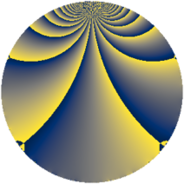# Properties

 Label 525.3.brLevel $525$ Weight $3$ Character orbit 525.br Rep. character $\chi_{525}(31,\cdot)$ Character field $\Q(\zeta_{30})$ Dimension $640$ Sturm bound $240$

# Related objects

## Defining parameters

 Level: $$N$$ $$=$$ $$525 = 3 \cdot 5^{2} \cdot 7$$ Weight: $$k$$ $$=$$ $$3$$ Character orbit: $$[\chi]$$ $$=$$ 525.br (of order $$30$$ and degree $$8$$) Character conductor: $$\operatorname{cond}(\chi)$$ $$=$$ $$175$$ Character field: $$\Q(\zeta_{30})$$ Sturm bound: $$240$$

## Dimensions

The following table gives the dimensions of various subspaces of $$M_{3}(525, [\chi])$$.

Total New Old
Modular forms 1312 640 672
Cusp forms 1248 640 608
Eisenstein series 64 0 64

## Trace form

 $$640 q + 160 q^{4} + 6 q^{5} - 4 q^{7} + 36 q^{8} - 240 q^{9} + O(q^{10})$$ $$640 q + 160 q^{4} + 6 q^{5} - 4 q^{7} + 36 q^{8} - 240 q^{9} + 30 q^{10} + 30 q^{11} - 60 q^{14} + 24 q^{15} + 320 q^{16} - 16 q^{22} - 108 q^{23} + 34 q^{25} + 196 q^{28} - 120 q^{29} + 192 q^{30} - 180 q^{31} + 48 q^{32} - 216 q^{33} - 164 q^{35} + 960 q^{36} + 28 q^{37} + 402 q^{38} - 42 q^{40} + 12 q^{42} - 920 q^{43} - 160 q^{44} + 18 q^{45} + 20 q^{46} + 420 q^{47} - 140 q^{49} + 108 q^{50} - 486 q^{52} - 324 q^{53} + 180 q^{56} + 192 q^{57} - 36 q^{58} - 720 q^{59} + 342 q^{60} + 180 q^{61} - 6 q^{63} - 500 q^{64} + 198 q^{65} + 416 q^{67} + 132 q^{68} + 498 q^{70} + 160 q^{71} + 54 q^{72} + 156 q^{73} - 540 q^{74} - 144 q^{75} - 60 q^{77} - 240 q^{78} + 80 q^{79} - 528 q^{80} + 720 q^{81} - 2316 q^{82} + 720 q^{84} - 1964 q^{85} - 240 q^{86} - 108 q^{87} - 1182 q^{88} - 430 q^{91} - 2172 q^{92} + 576 q^{93} + 480 q^{94} + 290 q^{95} - 1350 q^{96} + 488 q^{98} - 120 q^{99} + O(q^{100})$$

## Decomposition of $$S_{3}^{\mathrm{new}}(525, [\chi])$$ into newform subspaces

The newforms in this space have not yet been added to the LMFDB.

## Decomposition of $$S_{3}^{\mathrm{old}}(525, [\chi])$$ into lower level spaces

$$S_{3}^{\mathrm{old}}(525, [\chi]) \cong$$ $$S_{3}^{\mathrm{new}}(175, [\chi])$$$$^{\oplus 2}$$